# 【Python】用Python来训练数学计算？

【Python】用Python来训练数学计算？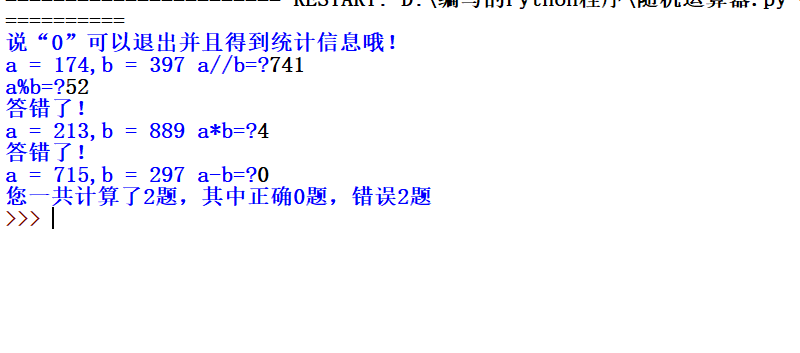# By Forty / Three % Two
import random
right = 0     # 正确回答数
kacha = 0     # 错误回答数
zongshu = 0   # 总回答数
print("说“0”可以退出并且得到统计信息哦！")
while True:
try:
fuhao = ["+","-","*","/"]      # 运算符号
a = round(random.uniform(1,1000))                          # 生成随机数
b = round(random.uniform(1,1000))                          # 生成随机数
c = random.choice(fuhao)                                   # 随机选择符号
if c == "+":    # 如果运算符号是+
temp = input("a = {},b = {} a+b=?".format(a,b))   # 获取回答
temp = int(temp)
zongshu += 1
if temp == a + b:
print("答对了！")
right += 1
elif temp == 0:
print("您一共计算了{}题，其中正确{}题，错误{}题".format(zongshu,right,kacha))
break
else:
print("答错了！")
kacha += 1

if c == "-":
temp = input("a = {},b = {} a-b=?".format(a,b))
temp = int(temp)
zongshu += 1
if temp == a - b:
print("答对了！")
right += 1
elif temp == 0:
print("您一共计算了{}题，其中正确{}题，错误{}题".format(zongshu,right,kacha))
break
else:
print("答错了！")
kacha += 1

if c == "*":
temp = input("a = {},b = {} a*b=?".format(a,b))
temp = int(temp)
zongshu += 1
if temp == a * b:
print("答对了！")
right += 1
elif temp == 0:
print("您一共计算了{}题，其中正确{}题，错误{}题".format(zongshu,right,kacha))
break
else:
print("答错了！")
kacha += 1

if c == "/":
temp = input("a = {},b = {} a//b=?".format(a,b))   # 获取商
temp = int(temp)
temp2 = input("a%b=?")                            # 获取余数
temp2 = int(temp)
if temp == a // b and temp2 == a % b:
print("答对了！")
right += 1
elif temp == 0:
print("您一共计算了{}题，其中正确{}题，错误{}题".format(zongshu,right,kacha))
break
else:
print("答错了！")
kacha += 1
except:
print("请输入合法的答案")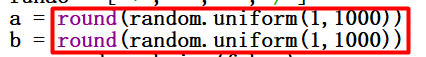（有些变量的意思我就不写了，大家可以看看代码里的注释）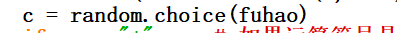PS：别忘了加上while循环和try哦！

try后面是要运行的内容，except后面是如果报错了，该怎么处理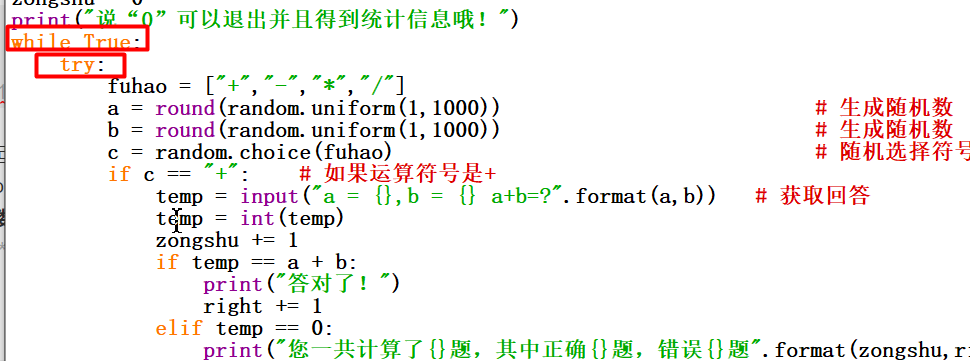（由于有四种运算符号，所以这里写了四个if，如果有更简便的方法欢迎指导）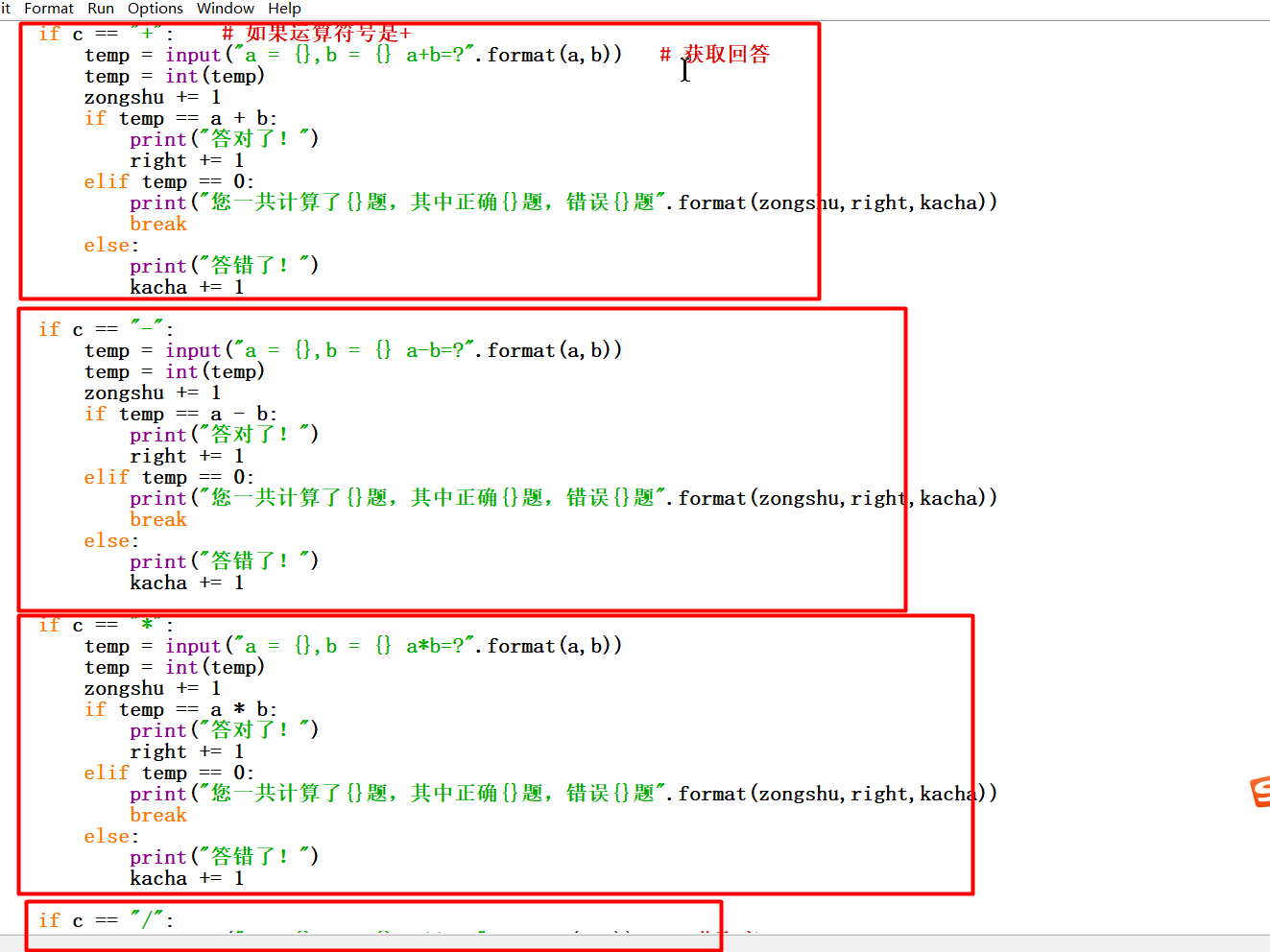0是退出，所以我们要加上break退出循环，并且打印结算信息。至于format的用法可以自行了解。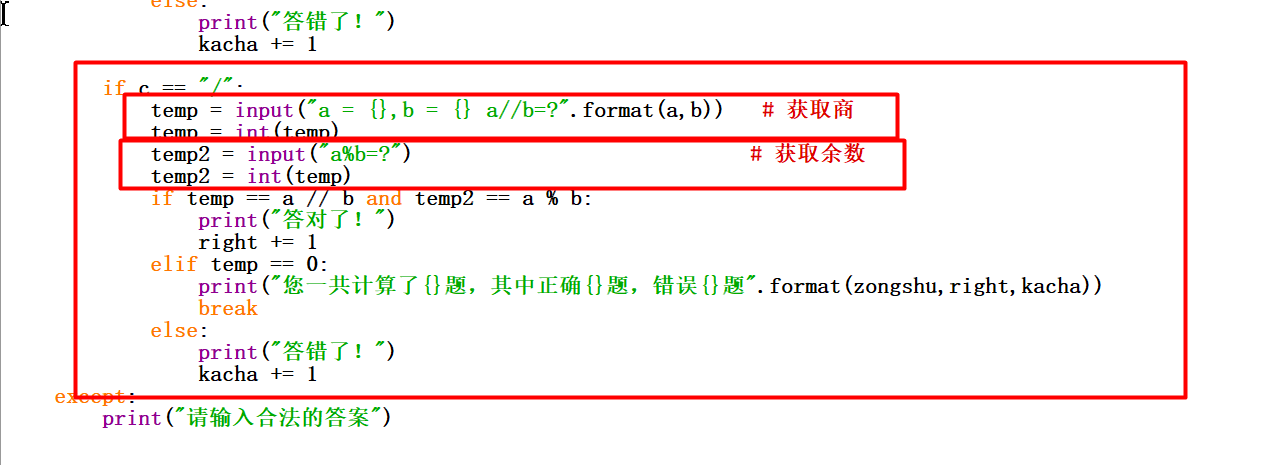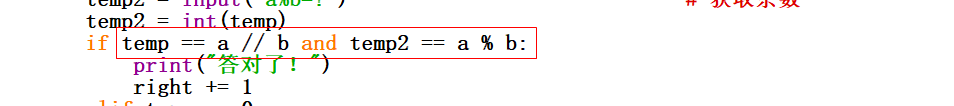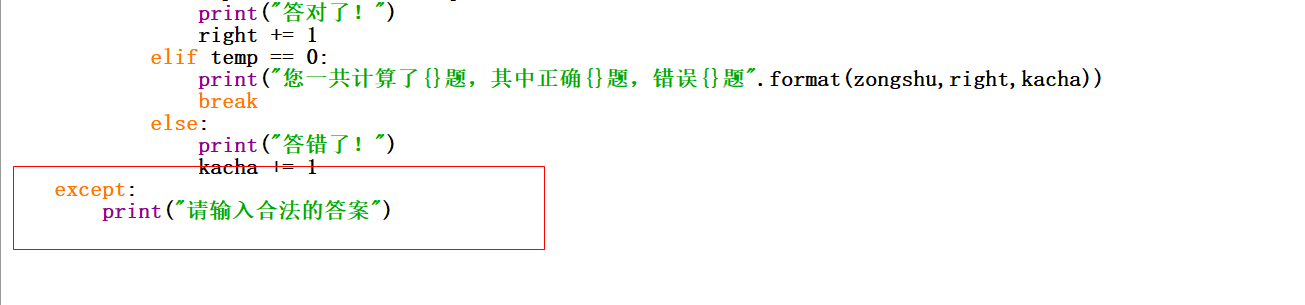©️2019 CSDN 皮肤主题: 游动-白 设计师: 上身试试